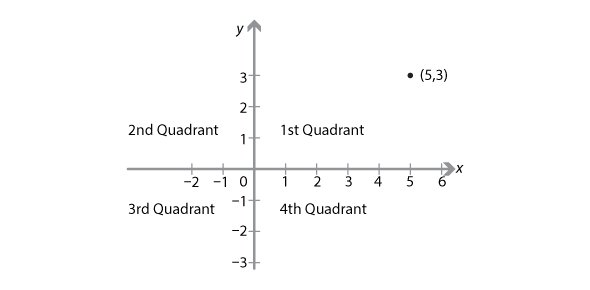# Coordinate Plane Assessment Test

10 Questions | Total Attempts: 1279SettingsCoordinate plane is a plane spanned by the x-axis and y-axis in which the coordinates of a point are its distances from two intersecting perpendicular axes. Take this assessment test to assess your knowledge of coordinate plane.

• 1.
The horizontal number line on a coordinate plane is known as the...
• A.

Y-axis

• B.

X-axis

• C.

Z-axis

• D.

W-axis

• 2.
The point at which the two axes intersects is called...
• A.

Origin

• B.

Base

• C.

Curve

• D.

Plane

• 3.
How many quadrants are in a coordinate plane?
• A.

4

• B.

3

• C.

2

• D.

5

• 4.
A flat surface that extends forever in two dimensions, but has no thickness is...
• A.

Board

• B.

Plane

• C.

Angle

• D.

Line

• 5.
Which of these is essential in finding a missing coordinate?
• A.

Slope

• B.

Number

• C.

Figure

• D.

Point

• 6.
In writing coordinates, which one comes first?
• A.

D

• B.

X

• C.

Y

• D.

B

• 7.
Which of the following can be used to find the distance between two points on a coordinate plane?
• A.

Compasseon

• B.

Pythagorean Theorem

• C.

Euclidean Geometry

• D.

Ruler's Rule

• 8.
A vector that starts at a point in the plane and has a direction that is perpendicular to the surface of the plane is referred to as...
• A.

Normal vector

• B.

Planar line

• C.

Linear curve

• D.

Slope

• 9.
A plane with x and y-axis defined is referred to as...
• A.

Triangle

• B.

Cartesian plane

• C.

Curve

• D.

Plot

• 10.
A point is often referred to as...
• A.

Dot

• B.

Zero dimension

• C.

Angle

• D.

Slope

Related Topics